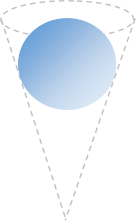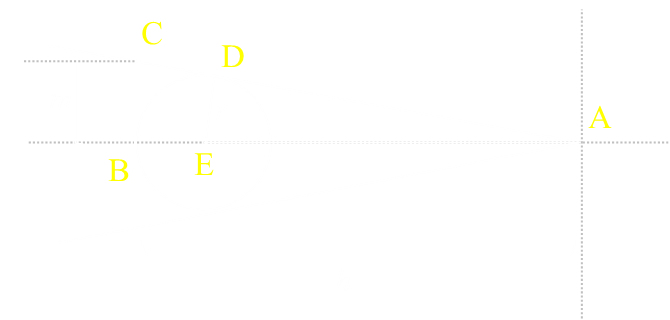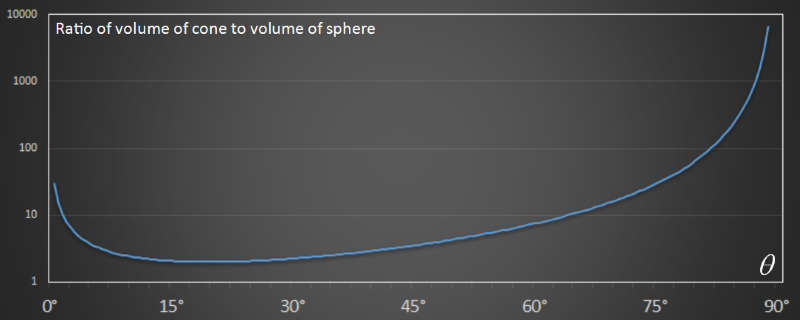﻿ Ice Cream Cones# Ice Cream ConesImage: George Oates Why are ice cream cones shaped the way they are? I never really thought about it much before. Sure, their conical shape makes them easy to hold, but is there any mathematical evidence for their shape? Could it be something to do with the efficiency about which they can hold ice cream?  CAVEAT  This article is a light hearted look at the mathematics involved in inserting spheres in cones. Outside of the math, I have zero practical, documented, or anecdotal evidence of any kind backing up this research! At no time have I contacted anyone in the ice cream or cone manufacturing industry for their input. It really is just an excuse to dust off a little Calculus. Take from this article what you will, it's just a bit of Summer fun …

### Ice cream

 Scooped balls of ice cream can be approximated as spheres. Ice cream cones can be approximated as hollow thin-walled circular base cones.What is the purpose of a cone? Its purpose is to efficiently hold your favorite ice cream (or frozen yoghurt, or sorbet). Efficient can mean many things, but I'm going to define efficiency to be the largest amount (volume) of ice cream relative to the volume of the cone. Specifically, if we want to put a sphere inside a cone, what configuration results in the ratio of the volume of the sphere to the volume of the cone being the highest? If you were to insert a spherical blob of ice cream into a cone, what shape cone will hold the most amount of delicious ice cream and the least amount of 'wasted' air? (Of course, after you have filled the cavity of the cone in the most efficient way possible, it's up to you to decide if you want to place another scoop on top!)
 The volume of a cone is easy to calculate (It's 1/3 of that of a cylinder of the same height). However, we need to account for the angle of the cone. Choose too narrow a cone, and there will be a lot of wasted space below the ice cream. Choose too broad a cone, and the wasted space is around the edge of the ice cream ball.To paraphrase The Three Bears: “This one is too tall. This one is too short. This one is juuuussttt right!” We need to find the one that is just right.

### Similar Triangles

Let's see if we can solve this with a little geometry:Our sphere rests inside the cone, and just touches the inside at one location forming a ring. A cross section shows this is tangential to the sphere (point D).

Δ ADE is a right angle triangle.

Δ ABC is also a right angle triangle, and they share the same angle at the top of the cone, so are similar triangles.

As the triangles are similar, the ratio of their sides is the same.This gives the equation for the radius of a sphere r that fits in a cone with height h, and circular end cap of radius m.

This is great, but not what we want. What we want to do is find the most efficient solution. We what to find out is what is the shape of the cone that has the minimum volume that can enclose a sphere of given size.

We're back to our old friend Calculus …### Minimum Volume

 Knowing that there are multiple solutions for cones that contain a sphere, what is the most efficient shaped cone? We'll use θ to describe the cone. The volume of the cone is:From geometry/trigonometry we can determine the relationship between the height, sphere radius, and angle of cone:From similar triangles (as we learned above), there is another way to describe the angle of the cone. We can then combine these two results to get an equation that describes the radius of the cone purely interms of the angle of the cone:We now have everything we need, and can plug this into the formula for the volume of the sphere:This is a fun little equation, so let's take some time to plot it out. Below is a graph showing the volume of the cone against the cone angle. I've normalized the graph by dividing the volume of the cone by that of the sphere to get a simple ratio.On the x-axis is plotted the cone angle from 0° on the left to 90° (π/2) on the right.

On the y-axis is the ratio of the two volumes (on a log scale).

When the cone is very sharp, pointy and trumpet like, with a very small angle, the cone has a high volume relative to the sphere (It's not defined at exactly 0°). As the cone angle increases, the ratio of volume falls to some minimum (which we'll calculate later), then increases again.

As the cone angle gets larger and larger, the cone flattens out, and the volume ratio increases again until at 90° it's infinitely wide.### Unleash the Calculus hounds …

Starting with our equation for the volume of the cylinder we can differentiate with respect to the cone angle. Setting this first derivative to zero allows us to find the turning points.This looks a little intimidating, but bear with me, things start to cancel out nicely. You knew that one day you'd find a use for all those trig equalities you were made to learn. Today could be that day …We're almost there. Just a little more expansion, grouping and factoring, and we're there:This is our very important result. It tells us what the roots of our equation are.

The root on the right is mathematically interesting, but meaningless in this context. For (sinθ + 1) = 0 means that sinθ = -1, which is not a real-world answer (we're looking for a value of theta that is acute in nature; less than 90°)The root on the left is far more interesting. It tells us the angle of the cone at the turning point of the function.

Substituting 1/3 into the earlier definition gives us the result:The most efficient cone, is one with a height of four times the radius of the sphere. The angle this occurs at is ≈ 19.47° The volume of the cone at this angle is exactly double the volume of the sphere.A result like this deserves sprinkles! If you choose your cone angle correctly you can ensure than your spherical ball of ice cream takes up 50% of the volume of the cone. The maximum possible.

## Real World Cones

I don't know about you, but looking at the picture below, that angle looks pretty close to 20° to me!Coincidence? I'll let you decide …

You can find a complete list of all the articles here.Click here to receive email alerts on new articles.